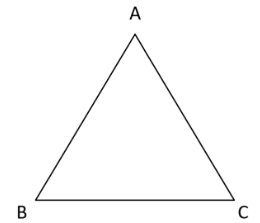QuestionAnswers

# The angles of a triangle are in the ratio $1:3:5$. Find the measure of each of the angles.

Hint: You know that the sum of angles of a triangle is ${180^ \circ }$. And if angles are given in ratio like $\angle A:\angle B:\angle C = 1:3:5$ you can write as
$\angle A = x,\angle B = 3x,\angle C = 5x$ and use the sum of angles $= {180^ \circ }$, you will get the answer.

So, basically, the question is saying that there is a triangle whose angles are in ratio $1:3:5$. And now we have to find each angle.
So first of all, let us assume that $ABC$ is a triangle.Now, it is given that angles are in ratio $1:3:5$
So, we can write $\angle A,\angle B,\angle C$ as the angles of $\vartriangle ABC$.
Now according to question, it is given that
$\angle A:\angle B:\angle C = 1:3:5$
As we know that if we remove the proportionality sign we must multiply by a constant.
Let $x$ be the constant
Then we can say that
$\angle A = 1 \times x \\ \angle B = 3 \times x \\ \angle C = 5 \times x \\$
So, we get all three angle in terms of $x$
That is, $\angle A = x,\angle B = 3x,\angle C = 5x$
So as we all know that the sum of angles of a triangle is ${180^ \circ }$.
i.e. $\angle A + \angle B + \angle C = {180^ \circ }$
Here we put the value of all three angles , we will get
$x + 3x + 5x = 180 \\ 9x = 180 \\ x = {20^ \circ } \\$
So now we can easily find the values of all the angles.
$\angle A = x = {20^ \circ } \\ \angle B = 3x = 3 \times 20 = {60^ \circ } \\ \angle C = 5x = 5 \times 20 = {100^ \circ } \\$
(As we know that $\angle A = x,\angle B = 3x,\angle C = 5x$)
Hence, ${20^ \circ },{60^ \circ },{100^ \circ }$ are the angles of the triangle.

Note: As it is given that $a:b$ it means $\dfrac{a}{b}$. So in this question, $\angle A:\angle B:\angle C = 1:3:5$.
This means, $\dfrac{{\angle A}}{{\angle B}} = \dfrac{1}{3},\dfrac{{\angle B}}{{\angle C}} = \dfrac{3}{5}$
So, $\angle A = x \\ \angle B = 3x \\ \angle C = \dfrac{5}{3}\angle B \\$
That is, $\angle C = \dfrac{5}{3} \times 3x = 5x$
Then we put in the equation of $\vartriangle ABC$
$\angle A + \angle B + \angle C = {180^ \circ }$
And we will get the required answer.